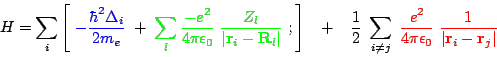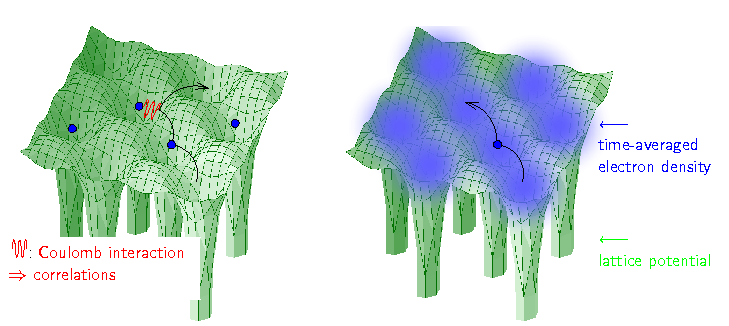Technische Universität Wien
Institut für Festkörperphysik

## Computational Materials Science

### Dynamical mean field theory and its extensions

Dynamical mean field theory (DMFT) and its numerous extensions represent a big step forward in our efforts to develop reliable methods for describing electronic correlations. Depending on the strength of the electronic correlation, these non-perturbative methods correctly yield a weakly correlated metal, a strongly correlated metal, or a Mott insulator. Applied to model Hamiltonians, DMFT was a big success and advanced our understanding of correlation effects like the Mott-Hubbard metal-insulator transition. More recently, extensions of DMFT such as the so-called quantum cluster theories as well as other extensions based on diagrammatic approaches have been quickly unveiling the missing aspects of the complete picture, where also non-local physics beyond DMFT is treated.

DMFT has been successfully merged with conventional bandstructure calculations in the local density approximation (LDA), thereby allowing for the realistic calculation of materials with strong electronic correlations such as transition metal oxides or heavy Fermion systems, which was hitherto not possible.

### Electronic Structure Calculations with DMFT

One of the most important challenges of theoretical physics is the development of tools for the accurate calculation of material properties. In solid state theory, we already know our ''theory of everything'', which consists of three terms: the kinetic energy, the lattice potential, and the Coulomb interaction between electrons:(1)

Here,anddenote the position of electronand ionwith chargeand, respectively,is the Laplace operator of the kinetic energy,andare the vacuum dielectric and Planck constant, see Fig. 1 for an illustration. Despite knowing the Hamiltonian, it is impossible to solve, even numerically, if more than a very few electrons are involved. This is due to the last term, the Coulomb interaction, which correlates the movement of every electronwith every other electron.

Since electrons have to be described quantum-mechanically, the numerical effort grows exponentially with the number of electrons.Figure 1:
Solid State Hamiltonian
To calculate a material, one has to take into account three terms: the kinetic energy favoring the electrons to move through the crystal (black), the lattice potential of the ions (green), and the Coulomb interaction between the electrons (red). Due to the latter, the first electron is repelled by the second, and it is energetically favorable to hop somewhere else, as depicted. Hence, the movement of every electron is correlated with that of every other, which prevents even a numerical solution.
LDA approximation
LDA is an approximation which allows to calculate material properties but which dramatically simplifies the electronic correlations: every electron moves independently, i.e., uncorrelated, within a time-averaged local density of the other electrons.

In this situation, one can either dramatically simplify the Hamiltonian (1), hoping that the simplified model allows for a qualitative understanding including correlation (many-body) effects, or employ equally dramatic approximations to deal with (1). These two paths have been followed by the two big communities of solid state theory, the many-body model-Hamiltonian and the density functional community.

Within density functional theory, the local density approximation (LDA) turned out to be unexpectedly successful, and established itself as the method for realistic solid state calculations in the last century. This is surprising because LDA is a serious approximation to the Coulomb interaction. Basically, an electron atsees a time-averaged density of the other electrons, with a corresponding local LDA potential, see Fig. 1. This reduces the problem to a single electron calculation.

The success of LDA shows, that this treatment is actually sufficient for many materials, both for calculating ground state energies and bandstructures, implying that electronic correlations are rather weak in these materials. But, there are important classes of materials where LDA fails, such as transition metal oxides or heavy fermion systems. In these materials the valence orbitals are theandorbitals. For two electrons in these orbitals the distanceis particularly short, and electronic correlations particularly strong.

Many such transition metal oxides are Mott insulators, where, the on-(lattice-)site Coulomb repulsionsplits the LDA bands into two sets of Hubbard bands. One can envisage the lower Hubbard band as consisting of all states with one electron on every lattice site and the upper Hubbard band as those states where two electrons are on the same lattice site. Since it costs an energyto have two electrons on the same lattice sites, the latter states are completely empty and the former completely filled with a gap of sizein-between, see Fig. 2. Other transition metal oxides and heavy fermion systems are strongly correlated metals, with heavy quasiparticles at the Fermi energy, described by an effective mass or inverse weight, see Fig. 2.Figure 2:
Weakly correlated metal
The on-site Coulomb interactionis weak compared to the LDA bandwidth; and LDA gives the correct answer, typically a (weakly correlated) metal for which we schematically draw a density of statesat energiesand the bandstructure (vs. wave vector).
Strongly correlated metal
In this intermediate regime, one has already Hubbard bands, like for(right panel), but at the same time a reminder of the weakly correlated LDA metal (left panel), in form of a quasiparticle peak: The () LDA bandstructure is reproduced, albeit with its width and weight reduced by a factorand life-time broadening effects.
Mott insulator
If the Coulomb interaction becomes large (), the LDA band splits into two Hubbard bands, and we have a Mott insulator with only the lower band occupied (at integer fillings); such a splitting can be described by the so-called LDAU method.
 The entire parameter regime and physics is described by LDADMFT

Dynamical mean-field theory (DMFT) is a modern, non-perturbative many-body technique, which describes such kind of correlation physics. It was first applied to model Hamiltonians - with big success. Hence, DMFT was recently merged with the conventional LDA approach for calculating materials with strong electronic correlations realistically. The first LDADMFT calculations turned out to be a breakthrough in this respect, which might not be astonishing as it is able to describe the full range of materials from weakly to strongly correlated metals to Mott insulators, see Fig. 2. LDADMFT was successful in calculating, among others, the-transition in cerium, the metal-insulator transition in VO, and the-phase of plutonium.

### Outlook

Electronic structure calculations with DMFT have just begun. Many important physical quantities such as the optical and thermal conductivity, magnetic and orbital susceptibilities, and thermodynamic properties have not yet been calculated or only for a single material. Many important transitions and the physics beyond have neither yet been studied with LDADMFT nor are they understood. Besides LDADMFT, other methods employing DMFT to do electronic structure calculations are presently developed. Generalizations to extend DMFT towards cluster algorithms, which can describe, e.g., d-wave superconductivity, have been established and have been already successfully applied to model Hamiltonians.